# HTML | DOM InputEvent

The input event is fired when the user changes an element, the value of an element or <textarea> element.
DOM InputEvent occurs when an element in the HTML document gets input from the user.

InputEvent property:

• data: Returns the inserted characters.
• dataTransfer: Returns an object containing information about the inserted/deleted data.
• getTargetRanges: Returns an array containing target ranges that will be affected by the insertion/.eletion.
• inputType: Returns the type of the change (i.e “inserting” or “deleting”)
• isComposing: Returns the state of the event.

Syntax:

```<element oninput="Function">
```

Example-1: Accessing input type using “event.inputType;”

 ` ` `<``html``> ` ` `  `<``body``> ` `    ``<``input` `type``=``"text"`  `           ``id``=``"myInput"`  `           ``oninput``=``"myFunction(event)"``> ` ` `  `    ``<``p``>The type of action:<``span` `id``=``"demo"``> ` `      `` ` ` `  `    ``<``script``> ` `        ``function myFunction(event) { ` `            ``document.getElementById( ` `              ``"demo").innerHTML = ` `              ``event.inputType; ` `        ``} ` `    `` ` ` `  ` ` ` `  ` `

Output:
Before: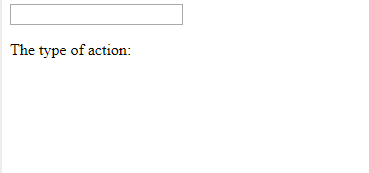After: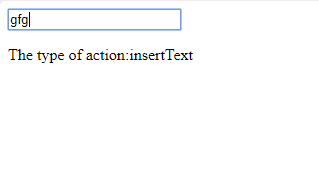Example-2: Accessing data property to return inserted characters.

 ` ` `<``html``> ` ` `  `<``body``> ` ` `  `    ``<``input` `type``=``"text"`  `           ``id``=``"myInput"`  `           ``oninput``=``"myFunction(event)"``> ` ` `  `    ``<``p``>The inserted character: <``span` `id``=``"demo"``> ` `      `` ` ` `  `    ``<``script``> ` `        ``function myFunction(event) { ` `            ``document.getElementById( ` `              ``"demo").innerHTML =  ` `              ``event.data; ` `        ``} ` `    `` ` ` `  ` ` ` `  ` `

Output:
Before: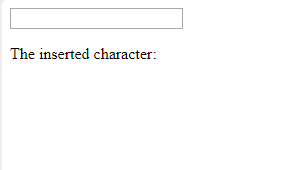After: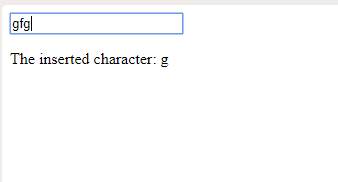Example-3: oninput return the whole inserted data.

 ` ` `<``html``> ` ` `  `<``body``> ` `    ``<``p``>Write something in the text  ` `      ``field to start the function.... ` `    ``<``input` `type``=``"text"`  `           ``id``=``"myInput"`  `           ``oninput``=``"Function()"``> ` ` `  `    ``<``p` `id``=``"demo"``> ` ` `  `    ``<``script``> ` `        ``function Function() { ` `            ``var x = document.getElementById( ` `              ``"myInput").value; ` `           `  `            ``document.getElementById( ` `              ``"demo").innerHTML =  ` `              ``"You wrote: " + x; ` `        ``} ` `    `` ` ` `  ` ` ` `  ` `

Output:
Before: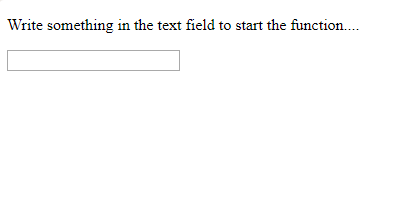After: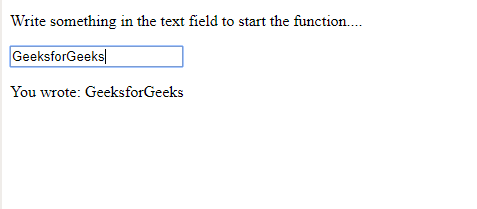Supported Browsers: